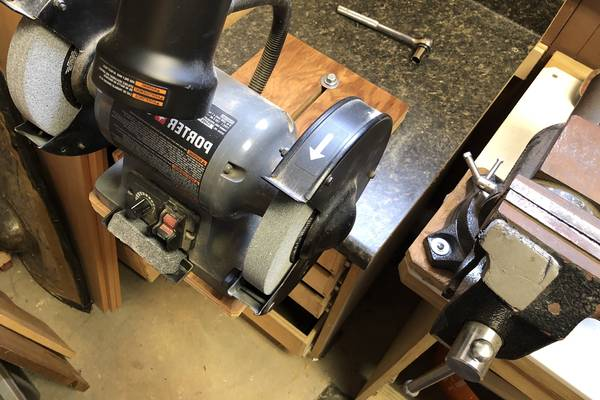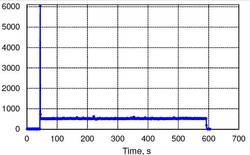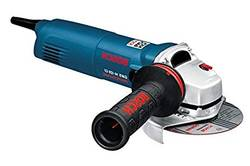# How Many Watts Does a Bench Grinder Use? (Wattage Options)

Sometimes it is all about power. Bench grinders are power tools and they need a constant supply of power to work correctly. But that may mean your electricity bill may go up each month, or will it. Studies have shown that bench grinders do not use as much electricity as angle grinders.

How many watts does a bench grinder use? The amount of watts used by a bench grinder varies. It will depend on its motor size and all the extras attached to it. Less powerful motors will use fewer watts. When the wheels are spinning freely and have no resistance, then it uses about 76 watts.

If you need to track your electrical use, then continue to read our article. We explore the power use of a variety of different bench grinders. The information contained here should help you find the right grinder for you and your electrical use limits.

## How Many Amps Does a Bench Grinder Use

To figure out how many amps your bench grinder uses at one time, you need to divide watts by volts. So if you have a 250-watt bench grinder, and it uses 110 volts, then the bench grinder is using.

The answer to that problem is the 250-watt bench grinder will use just over 2 amps. Don’t think about HP. The number of HP is not a key number and does not factor into the calculations at all.

## Power Consumption of GrinderThe general rule about power consumption is that it is not about the power of the motor, etc. But how much electrical energy is consumed over time. So if you rarely use your bench grinder, then it does not consume that much power.

On the other hand, if you use your bench grinder a couple of hours each day, 6 days a week, then it will consume a lot of power. One of the problems that enters into this is that power tools and other power appliances are not 100% efficient.

They will consume far more power than they need to use. Power is like heat, it is wasted when you use a power tool like a bench grinder. Here is what power consumption you can expect from the following bench grinders:

• 1. 150-watt bench grinder - This size of bench grinder will use about 1.1 amps.
• 2. 500-watt bench grinder - When you upgrade to this size of bench grinder then you are using about 4 amps of power.
• 3, 750-watt bench grinder - this much power raises your amp use to 6 1/4 amps.
• 4. 900-watt bench grinder - the more powerful the power tool, the more amps you will use. At this level, you are going to use 7 1/2 amps of power.

## Understanding Amps

When you want to measure electrical current, you look to amps to do the job, For example, if you have a battery that advertises that it has 20 amp capacity, then it will supply that 20 amps in a variety of ways.

You can use 1 amp per hour for 20 hours, or you can use 20 amps for 1 hour. It all depends on how much electrical current you want at the time. To figure out how many kilowatt-hours you used, you multiply the amps by the voltage, then divide by 1000.

That should give you your power consumption in a nutshell.

## Cost by Watts Used

A 500-watt bench grinder doesn’t use 500 watts all the time it is on. When the wheels are spinning freely and have no resistance, then it uses about 76 watts to spin those wheels. But when you add resistance, then while grinding, the bench grinder will use roughly 500 watts.

500 watts used equals about .50 kilowatt-hours. This figure translates into .01 cents per minute or 6.1 cents every hour, 49 cents per day. At the end of the month, if you use your bench grinder every day, all day, your electric bill will go up \$9.31.

## Angle Grinder WattageThis handy power tool has a far more powerful motor in it and it can use up to 1535 watts every time you use it. This number makes it far more expensive to use than a bench grinder.

1535 watts equals 1.535 kilowatt-hours. At current rates, you are looking at .31 cents per minute, 18.4 cents every hour, and \$1.47 per day. If you use the angle grinder frequently, then your electrical bill could go up by \$28.01 every month.

That is 3 times the expense of a bench grinder. Angle grinders have their purpose and their advantages but not when it comes to saving you money. They are far more expensive to run than a bench grinder.

### 1. How many amps equal one kilowatt-hour?

While the two measurements are not interchangeable, more data is required. You need to multiply the amps by the volts used (120) and then divide by 1000. Roughly 9 amps equals one kilowatt-hour.

### 2. How do I change amps into watts?

All you do is take the number of amps and multiply it by the number of volts. If you have a 12-volt battery and it produces 100 amps, then you multiply 12 by 1000. The number of watt-hours is 1200.